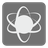Chemical Analysis
Undefined
English RU
The mission is in Blocked Mode. Access to the solutions is blocked for a day or two (even after you share your own), until we'll have enough solutions for you to check. All users who've solved the mission will get the notifications about their opening.

As the input you will get the chemical formula and the number N. Your task is to create a list of the chemical elements, which are in the formula in the amount of >= N.
Pay attention, that in the some formulas will be used brackets - () and []. This article will help you to open the brackets and don't make mistake while counting.

Input: Chemical formula and the number of atoms.

Output: List of the chemical elements, sorted in the alphabetical order.

Example:

```atoms('C2H5OH', 2) == ['C', 'H']
atoms('H2O', 2) == ['H']
atoms('Mg(OH)2', 1) == ['H', 'Mg', 'O']
atoms('K4[ON(SO3)2]2', 4) == ['K', 'O', 'S']
```

How it is used: For chemical analysis.

Precondition:
1 <= different elements <= 10